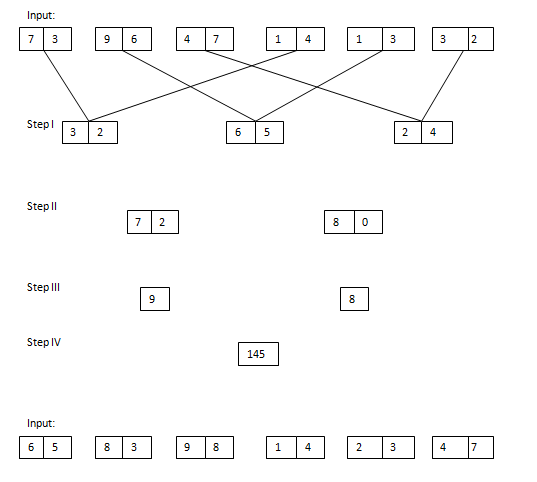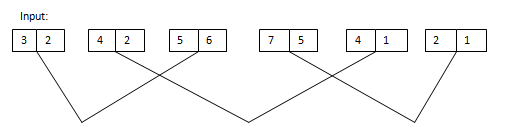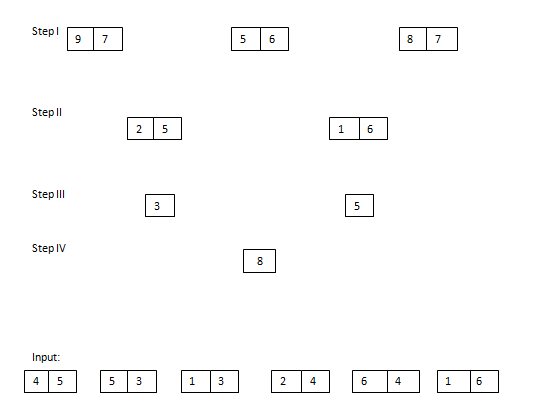# Important Questions BASED on NEW Pattern (Day-25): Reasoning(Input Output):

Important Questions BASED on NEW Pattern (Day-25): Reasoning(Input Output):

Dear Readers, IBPS Examinations was approaching shortly and many of our followers were requesting us to provide Practice Questions based on the new pattern, for that here WE have started providing Practice Questions Based on New pattern from Aptitude, Reasoning and English each one topic daily. Kindly follow us regularly and make use of it, if you have any suggestion kindly use the comment section below.

[WpProQuiz 622]

Directions (1-5): Study the following information carefully and answer the givenquestions.

A number arrangement machine arranges two digit numbers into a typical manner. Each step gives output taking input from the previous step. The following is an illustration of Input and rearrangement. Using the illustration answer the question given below.1.Which of the following combination represent the first digit of the third number and second digit of the first number in step I of the given input?

1. 1, 4
2. 2, 5
3. 1, 2
4. 5, 4
5. 2, 4

1. If first number is divided by second number in step II, then what will be the answer?
1. 1.75
2. 2.5
3. 1.5
4. 1.25
5. 3

3.What is the multiplication of two numbers obtained in step III?

1. 20
2. 24
3. 32
4. 48
5. 50

1. If the value ‘6^2’ is subtracted from the final output, then what will be the resultant value?
1. 9
2. 5
3. 4
4. 2
5. 0

5.Which of the following represent the difference between the first digit of the second number and second digit of the first number in step I?

1. 5
2. 3
3. 1
4. 4
5. 2

Directions (6-10): Study the following information carefully and answer the givenquestions.

A number arrangement machine arranges two digit numbers into a typical manner. Each step gives output taking input from the previous step. Thefollowing is an illustration of Input and rearrangement. Using the illustrationanswer the question given below.6.What is the addition of first number and third number in step I?

1. 161
2. 158
3. 144
4. 185
5. 198

7.Which of the following represent the difference between the first digit of the second number and second digit of the first number in step II?

1. 1
2. 2
3. 3
4. 4
5. 5

1. If the first and second numbers are square in step II, then what is the difference of both numbers in step II?
1. 452
2. 385
3. 421
4. 258
5. 369

1. If the value ‘5^2’ is multiplied with the final output, then what will be the resultant value?
1. 75
2. 50
3. 200
4. 100
5. 150

10.Which of the following combination represent the first digit of the third number and second digit of the first number in step I of the given input?

1. 9, 7
2. 6, 9
3. 8, 6
4. 7, 9
5. 9, 8
[WpProQuiz 622]